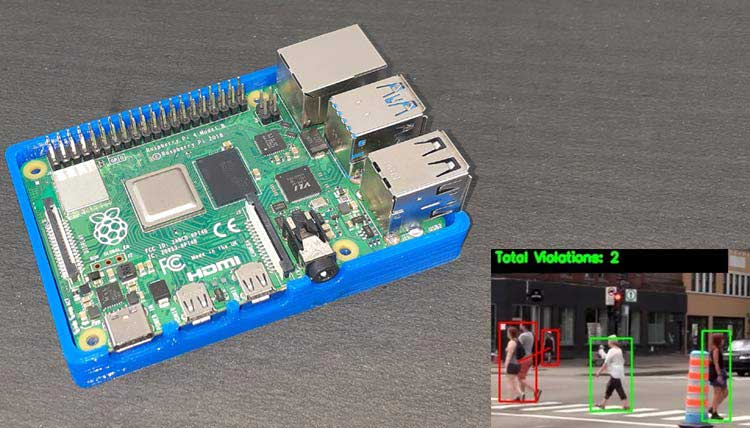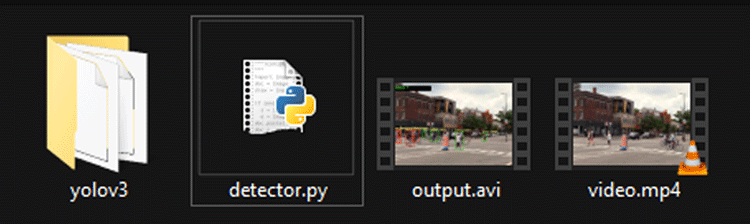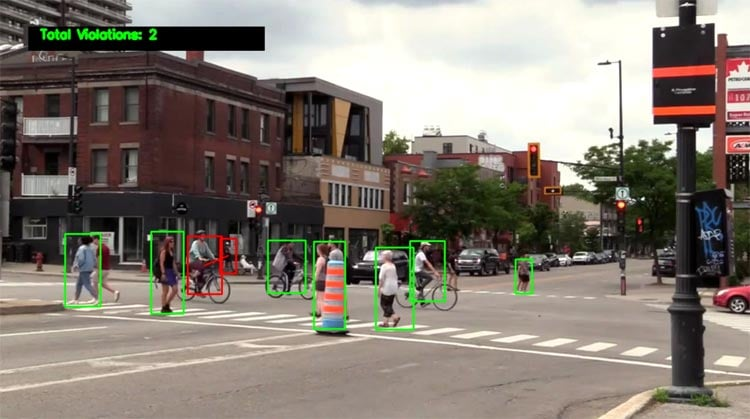# Social Distancing Detector Using OpenCV and Raspberry Pi

Published  September 21, 2020   10
A
AuthorIn the time of Covid-19, Social-distancing is an effective way to slow down the transmission of infectious virus. People are advised to minimize their contact with each other to minimize the risk of the disease being transmitted through direct contact. Maintaining a safe distance is a challenge for many places like factories, banks, buses or railway stations, etc.

So in continuation of our previous Corona safety projects like Automatic sanitizer machine and contactless temperature monitoring, here we are going to build a Social Distancing Detector system using OpenCV and Raspberry Pi. We’ll be using the weights of the YOLO v3 Object Detection Algorithm with the Deep Neural Network module.

Raspberry Pi is always a good choice for Image processing projects as it has more memory and speed than other controllers. We previously used Raspberry Pi for some complex image processing projects like facial landmark detection and Face recognition application

### Components Required

• Raspberry Pi 4

Here we only need RPi 4 with OpenCV installed on it. OpenCV is used here for digital image processing. The most common applications of Digital Image Processing are object detectionFace Recognition, and people counter.

### YOLO

YOLO (You Only Look Once) is a smart Convolution neural network (CNN) for real-time Object Detection. YOLOv3, the latest variant of the object detection algorithm, YOLO can recognize 80 different objects in images and videos, and it is super fast and has excellent accuracy. The algorithm applies a single neural network to the whole image, then separates the image into regions and calculates boundary boxes and probabilities for each area. Base YOLO model can process images in real-time at 45 frames per second. YOLO model outperforms all other detection methods like SSD and R-CNN.

The YOLOV3 model that we are going to use in this project can be downloaded from here.

### Installing OpenCV in Raspberry Pi

Before installing the OpenCV and other dependencies, the Raspberry Pi needs to be fully updated. Use the below commands to update the Raspberry Pi to its latest version:

`sudo apt-get update`

Then use the following commands to install the required dependencies for installing OpenCV on your Raspberry Pi.

```sudo apt-get install libhdf5-dev -y
sudo apt-get install libhdf5-serial-dev –y
sudo apt-get install libatlas-base-dev –y
sudo apt-get install libjasper-dev -y
sudo apt-get install libqtgui4 –y
sudo apt-get install libqt4-test –y```

Finally, install the OpenCV on Raspberry Pi using the below commands.

`pip3 install opencv-contrib-python==4.1.0.25`

If you are new to OpenCV, check our previous OpenCV tutorials with Raspberry pi:

We have also created a series of OpenCV tutorials starting from the beginner level.

### Installing other Required Packages in Raspberry Pi

Before programming the Raspberry Pi for Social distance detector, let’s install the other required packages.

Installing imutils: imutils is used to make essential image processing functions such as translation, rotation, resizing, skeletonization, and displaying Matplotlib images easier with OpenCV. Use the below command to install the imutils:

`pip3 install imutils`

### Program Explanation

Complete code is given at the end of the page. Here we are explaining the important sections of the code for a better explanation.

So at the starting of the code, import all the required libraries that are going to be used in this project.

```import numpy as np
import cv2
import imutils
import os
import time```

The Check() function is used to calculate the distance between two objects or two points in a frame of video. The points a and b denote the two objects in the frame. These two points are used to calculate the Euclidean Distance between the objects.

```def Check(a,  b):
dist = ((a - b) ** 2 + 550 / ((a + b) / 2) * (a - b) ** 2) ** 0.5
calibration = (a + b) / 2
if 0 < dist < 0.25 * calibration:
return True
else:
return False
```

The setup function is used to set the paths for the YOLO weights, cfg file, COCO names file. os.path module is used for common pathname manipulation. os.path.join() module is a sub-module of os.path and used to join one or more path components intelligently. cv2.dnn.readNetFromDarknet() method is used to load the saved weights into the network. After loading the weights, extract the list of all layers used in a network using a net.getLayerNames model.

```def Setup(yolo):
global neural_net, ln, LABELS
weights = os.path.sep.join([yolo, "yolov3.weights"])
config = os.path.sep.join([yolo, "yolov3.cfg"])
labelsPath = os.path.sep.join([yolo, "coco.names"])
ln = neural_net.getLayerNames()
ln = [ln[i - 1] for i in neural_net.getUnconnectedOutLayers()]```

Inside the image processing function, we take a single frame of video and then process it for social distancing detection between every person in-crowd. In the first two lines of the function, we set the dimensions of the video frame (W, H) as (None, None) initially. In the next line, we used cv2.dnn.blobFromImage() method to load frames in a batch and run them through the network. The blob function performs Mean subtraction, Scaling, and Channel swapping on a frame.

```(H, W) = (None, None)
frame = image.copy()
if W is None or H is None:
(H, W) = frame.shape[:2]
blob = cv2.dnn.blobFromImage(frame, 1 / 255.0, (416, 416), swapRB=True, crop=False)
neural_net.setInput(blob)
starttime = time.time()
layerOutputs = neural_net.forward(ln)```

The layer outputs from YOLO consist of a set of values. These values help us define which object belongs to which class. We loop over every output in the layerOutputs and as we are detecting people, we set the class label as "person". From each detection, we get a bounding box that gives us X center, Y center, Width, and Height of the box for detection in the output:

```            scores = detection[5:]
maxi_class = np.argmax(scores)
confidence = scores[maxi_class]
if LABELS[maxi_class] == "person":
if confidence > 0.5:
box = detection[0:4] * np.array([W, H, W, H])
(centerX, centerY, width, height) = box.astype("int")
x = int(centerX - (width / 2))
y = int(centerY - (height / 2))
outline.append([x, y, int(width), int(height)])
confidences.append(float(confidence))```

After that, calculate the distance between the center of the current box with all the other detected boxes. If the bounding boxes are close, then change the status to true.

```for i in range(len(center)):
for j in range(len(center)):
close = Check(center[i], center[j])
if close:
pairs.append([center[i], center[j]])
status[i] = True
status[j] = True
index = 0```

In the next lines, draw a rectangle around the person using the box dimensions we received from the model, then check if the box is safe or unsafe. If the distance between the boxes is close, then the box colour will be coloured red else the box will be coloured green.

```            (x, y) = (outline[i], outline[i])
(w, h) = (outline[i], outline[i])
if status[index] == True:
cv2.rectangle(frame, (x, y), (x + w, y + h), (0, 0, 150), 2)
elif status[index] == False:
cv2.rectangle(frame, (x, y), (x + w, y + h), (0, 255, 0), 2)```

Now inside the loop function, we are reading every frame of the video and then processing each frame to calculate the distance between the persons.

```ret, frame = cap.read()
if not ret:
break
current_img = frame.copy()
current_img = imutils.resize(current_img, width=480)
video = current_img.shape
frameno += 1
if(frameno%2 == 0 or frameno == 1):
Setup(yolo)
ImageProcess(current_img)
Frame = processedImg```

In the next lines, use the cv2.VideoWriter() function to store the output video at the location specified by opname which we have defined earlier.

```if create is None:
fourcc = cv2.VideoWriter_fourcc(*'XVID')
create = cv2.VideoWriter(opname, fourcc, 30, (Frame.shape, Frame.shape), True)
create.write(Frame)```

### Testing the Social Distance Detector Project

Once your code is ready, open up a Pi terminal and navigate to the project directory. The code, Yolo model, and the demo video should be in the same folder as shown below.You can download the YoloV3 directory from here, videos from Pexels and copy the Python code given below, and put them in the same directory as shown above.

Once you are in the project directory, execute the following command to start the code:

`python3 detector.py`

I tried this code on a video example that was obtained from Pexels. For me, the FPS was very slow and it took approx 10 to 11 minutes to process the entire video.Instead of using a video, you can even test this code with a Raspberry Pi Camera by replacing the cv2.VideoCapture(input) with cv2.VideoCapture(0) in 98th line of the code. Learn more about using PiCamera with Raspberry Pi by following the link.

This is how you can use OpenCV with Raspberry Pi to detect the social distancing violations. The output video and code is given below:

Code
```import numpy as np
import cv2
import imutils
import os
import time
def Check(a,  b):
dist = ((a - b) ** 2 + 550 / ((a + b) / 2) * (a - b) ** 2) ** 0.5
calibration = (a + b) / 2
if 0 < dist < 0.25 * calibration:
return True
else:
return False
def Setup(yolo):
global net, ln, LABELS
weights = os.path.sep.join([yolo, "yolov3.weights"])
config = os.path.sep.join([yolo, "yolov3.cfg"])
labelsPath = os.path.sep.join([yolo, "coco.names"])
ln = net.getLayerNames()
ln = [ln[i - 1] for i in net.getUnconnectedOutLayers()]
def ImageProcess(image):
global processedImg
(H, W) = (None, None)
frame = image.copy()
if W is None or H is None:
(H, W) = frame.shape[:2]
blob = cv2.dnn.blobFromImage(frame, 1 / 255.0, (416, 416), swapRB=True, crop=False)
net.setInput(blob)
starttime = time.time()
layerOutputs = net.forward(ln)
stoptime = time.time()
print("Video is Getting Processed at {:.4f} seconds per frame".format((stoptime-starttime)))
confidences = []
outline = []
for output in layerOutputs:
for detection in output:
scores = detection[5:]
maxi_class = np.argmax(scores)
confidence = scores[maxi_class]
if LABELS[maxi_class] == "person":
if confidence > 0.5:
box = detection[0:4] * np.array([W, H, W, H])
(centerX, centerY, width, height) = box.astype("int")
x = int(centerX - (width / 2))
y = int(centerY - (height / 2))
outline.append([x, y, int(width), int(height)])
confidences.append(float(confidence))
box_line = cv2.dnn.NMSBoxes(outline, confidences, 0.5, 0.3)
if len(box_line) > 0:
flat_box = box_line.flatten()
pairs = []
center = []
status = []
for i in flat_box:
(x, y) = (outline[i], outline[i])
(w, h) = (outline[i], outline[i])
center.append([int(x + w / 2), int(y + h / 2)])
status.append(False)
for i in range(len(center)):
for j in range(len(center)):
close = Check(center[i], center[j])
if close:
pairs.append([center[i], center[j]])
status[i] = True
status[j] = True
index = 0
for i in flat_box:
(x, y) = (outline[i], outline[i])
(w, h) = (outline[i], outline[i])
if status[index] == True:
cv2.rectangle(frame, (x, y), (x + w, y + h), (0, 0, 150), 2)
elif status[index] == False:
cv2.rectangle(frame, (x, y), (x + w, y + h), (0, 255, 0), 2)
index += 1
for h in pairs:
cv2.line(frame, tuple(h), tuple(h), (0, 0, 255), 2)
processedImg = frame.copy()
create = None
frameno = 0
filename = "newVideo.mp4"
yolo = "yolov3/"
opname = "output2.avi"
cap = cv2.VideoCapture(filename)
time1 = time.time()
while(True):
if not ret:
break
current_img = frame.copy()
current_img = imutils.resize(current_img, width=480)
video = current_img.shape
frameno += 1
if(frameno%2 == 0 or frameno == 1):
Setup(yolo)
ImageProcess(current_img)
Frame = processedImg
cv2.imshow("Image", Frame)
if create is None:
fourcc = cv2.VideoWriter_fourcc(*'XVID')
create = cv2.VideoWriter(opname, fourcc, 30, (Frame.shape, Frame.shape), True)
create.write(Frame)
if cv2.waitKey(1) & 0xFF == ord('s'):
break
time2 = time.time()
print("Completed. Total Time Taken: {} minutes".format((time2-time1)/60))
cap.release()
cv2.destroyAllWindows()```
Video

Tags

### problem

hi, after executing python3 detector.py I get a message that it was completed + time taken etc. However no video output or anything just the message. What might be the problem?

### How I can run the project

How I can run the project using Terminal?

### I am getting error when i run

I am getting error when i run the program from vnc server like :

Traceback (most recent call last):
File "detector.py", line 95, in <module>
Setup(yolo)
File "detector.py", line 18, in Setup
NotADirectoryError: [Errno 20] Not a directory: 'yolov3//coco.names'

### Geri izleme (en son çağrı):

Geri izleme (en son çağrı):

"Detektör.py" dosyası, satır 95, <module>

Kurulum (yolo)

Dosyasında "Detektör.py", 18. satır,

LABELS Kurulumunda = open (labelsPath) .read (). Strip () .split ("/ n")

NotADirectoryError: [Errno 20] Bir dizin değil: 'yolov3 // coco.names'

hatası alıyorum ve output.avi yi nasıl oluşturuyoruz rica etsem detaylı anlatabilir misiniz?

### Hello! Amazing work..

Hello! Amazing work..
But I have couple of questions about the check function, what 550 stands for? and also the 0.25 when comparing the distance?  how did you find it? I mean what are the parameters that you calculated these values based on?
Thank you so much! Hope you answer soon

### I am getting error when i run

I am getting error when i run the program from vnc server like :

Traceback (most recent call last):

File "detector.py", line 95, in <module>

Setup(yolo)

File "detector.py", line 18, in Setup